GFG App
Open AppBrowser
Continue

# Joining Data in R with Dplyr Package

In this article, we will be looking at the different methods of joining data with the dplyr in the R programming language.

We need to load the dplyr package. Type the below commands –

```Install - install.packages("dplyr")

## Method 1: Using  inner join

In this method of joining data,  the user call the inner_join function, which will result to jointed data with the records that have matching values in both tables in the R programming language.

inner_join() function:

This function includes all rows in `x` and `y`.

Syntax:

inner_join(x, y, by = NULL, on = NULL)

Parameters:

• x: A data.table
• y: A data.table
• by: A character vector of variables to join by.
• on: Indicate which columns in x should be joined with which columns in y.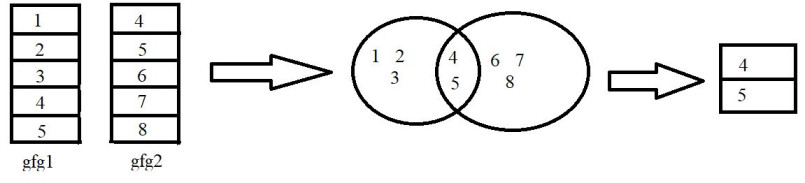Example:

In this example, we will be using the inner_join() function from the dplyr package to join two different data as shown in the image above in the R programming language.

## R

 `# load the library ` `library``(``"dplyr"``) ` ` `  `# create dataframe with 1 to 5 integers ` `gfg1 < -``data.frame``(ID=``c``(1: 5)) ` ` `  `# create dataframe with 4 to 8 integers ` `gfg2 < -``data.frame``(ID=``c``(4: 8)) ` ` `  `# perform inner join ` `inner_join``(gfg1, gfg2, by=``"ID"``) `

Output:

``` ID
1 4
2 5 ```

## Method 2: Using  left join

In this method of joining data,  the user call the left_join function and this will result to jointed data consisting of matching all the rows in the first data frame with the corresponding values on the second.s in the R programming language.

left_join() function:

This function includes all rows in `x`.

Syntax:

left_join(x, y, by = NULL, on = NULL)

Parameters:

• x: A data.table
• y: A data.table
• by: A character vector of variables to join by.
• on: Indicate which columns in x should be joined with which columns in y.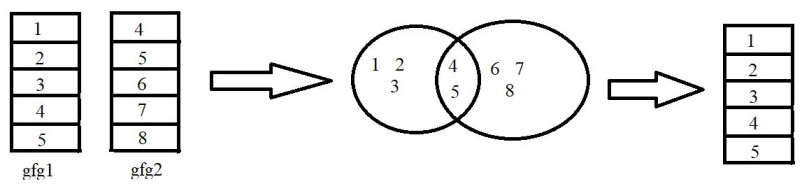Example:

In this example, we will be using the left_join() function from the dplyr package to join two different data as shown in the image above in the R programming language.

## R

 `# load the library ` `library``(``"dplyr"``)  ` ` `  `# create the dataframes ` `gfg1<-``data.frame``(ID=``c``(1:5)) ` ` `  `gfg2<-``data.frame``(ID=``c``(4:8)) ` ` `  `# perform left join ` `left_join``(gfg1,gfg2, by = ``"ID"``)     `

Output:

```  ID
1  1
2  2
3  3
4  4
5  5```

## Method 3: Using  right join

In this method of joining data, the user call the right_join function and this will result to jointed data consisting of matching all the rows in the second data frame with the corresponding values on the first in the R programming language.

right_join() function:

This function includes all rows in `y` and corresponding rows of ‘x’.

Syntax:

right_join(x, y, by = NULL, on = NULL)

Parameters:

• x: A data.table
• y: A data.table
• by: A character vector of variables to join by.
• on: Indicate which columns in x should be joined with which columns in y.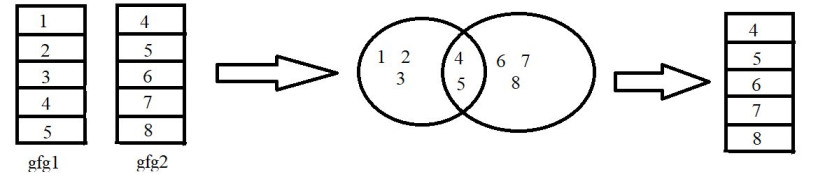Example:

In this example, we will be using the right_join() function from the dplyr package to join two different data as shown in the image above in the R programming language.

## R

 `# load the library ` `library``(``"dplyr"``)  ` ` `  `# create dataframes ` `gfg1<-``data.frame``(ID=``c``(1:5)) ` ` `  `gfg2<-``data.frame``(ID=``c``(4:8)) ` ` `  `# perform right join ` `right_join``(gfg1,gfg2, by = ``"ID"``)     `

Output:

```  ID
1  4
2  5
3  6
4  7
5  8```

## Method 4: Using full join

In this method of joining data,  the user calls the right_join function and  this will result in jointed data  of all the rows from the joined tables,

full_join() function:

This function includes all rows.

Syntax:

full_join(x, y, by = NULL, on = NULL)

Parameters:

• x: A data.table
• y: A data.table
• by: A character vector of variables to join by.
• on: Indicate which columns in x should be joined with which columns in y.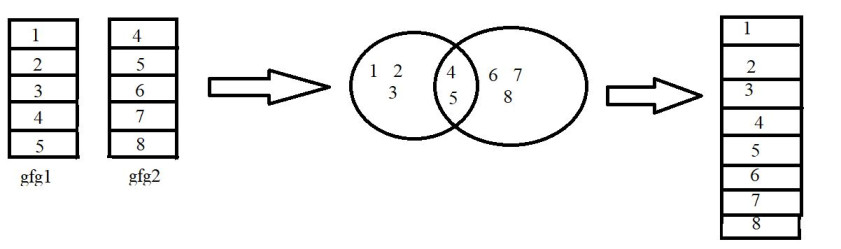Example:

In this example, we will be using the full_join() function from the dplyr package to join two different data as shown in the image above in the R programming language.

## R

 `# load library ` `library``(``"dplyr"``)   ` ` `  `# create dataframe ` `gfg1<-``data.frame``(ID=``c``(1:5)) ` `gfg2<-``data.frame``(ID=``c``(4:8)) ` ` `  `# perform full join ` `full_join``(gfg1,gfg2, by = ``"ID"``)     `

Output:

```  ID
1  1
2  2
3  3
4  4
5  5
6  6
7  7
8  8```

## Method 5: Using Semi join

In this method of joining data, the user calls the right_join function and this will return one copy of each row in the first table for which at least one match is found.

semi_join() function:

This function returns all rows from x where there are matching values in y, keeping just columns from x..

Syntax:

semi_join(x, y, by = NULL, on = NULL)

Parameters:

• x: A data.table
• y: A data.table
• by: A character vector of variables to join by.
• on: Indicate which columns in x should be joined with which columns in y.Example:

In this example, we will be using the semi_join() function from the dplyr package to join two different data as shown in the image above in the R programming language.

## R

 `# load the library ` `library``(``"dplyr"``)  ` ` `  `# create the dataframes ` `gfg1<-``data.frame``(ID=``c``(1:5)) ` `gfg2<-``data.frame``(ID=``c``(4:8)) ` ` `  `# perform semijoin ` `semi_join``(gfg1,gfg2, by = ``"ID"``)     `

Output:

```  ID
1  4
2  5```

## Method 6:  Using anti join

In this method of joining data, the user calls the right_join function and this will return all rows from x where there are no matching values in y, keeping just columns from x.

anti_join() function:

This function returns all rows from x where there are no matching values in y, keeping just columns from x.

Syntax:

anti_join(x, y, by = NULL, on = NULL)

Parameters:

• x: A data.table
• y: A data.table
• by: A character vector of variables to join by.
• on: Indicate which columns in x should be joined with which columns in y.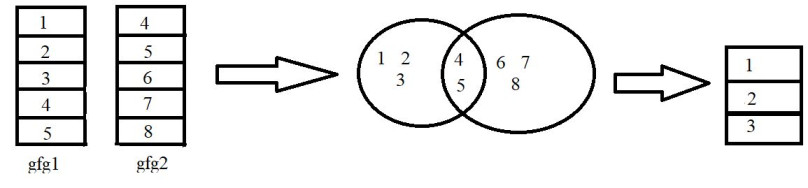Example:

In this example, we will be using the anti_join() function from the dplyr package to join two different data as shown in the image above in the R programming language.

## R

 `# load the library ` `library``(``"dplyr"``)  ` ` `  `# create the dataframes ` `gfg1<-``data.frame``(ID=``c``(1:5)) ` `gfg2<-``data.frame``(ID=``c``(4:8)) ` ` `  `# perform anti join ` `anti_join``(gfg1,gfg2, by = ``"ID"``)     `

Output:

```  ID
1  1
2  2
3  3```

My Personal Notes arrow_drop_up